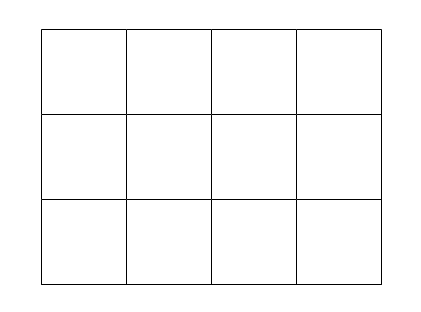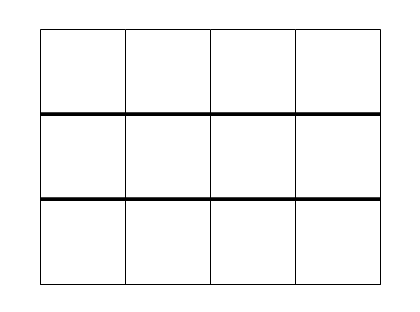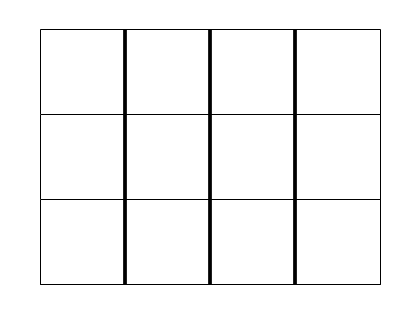Home > Patterns > Misunderstandings > Rectangular grids

# Rectangular grids

This rectangular grid is filled with 12 identical squares, with no spaces between them.A 3 $$\times$$ 4 rectangular grid.

That same rectangular grid can be seen as three rows of four squares.The three rows of the grid.

It can also be seen as four columns of three squares.The four columns of the grid.

A rectangular grid is therefore a repeating pattern in two ways.

The rectangular grid can be a most valuable aid in early mathematics learning.

• It incorporates many spatial concepts (square, rectangle, congruence, collinearity, parallels and perpendiculars).
• It can provide an introduction to multiplication.
• Counting the number of squares leads to skip counting (4, 8, 12 and 3, 6, 9, 12 in the above grid).
• Counting the number of squares also leads to multiplication (4 $$\times$$ 3 = 12 and 3 $$\times$$ 4 = 12).
• This counting eventually leads to the formula for the area of a rectangle.
• It can be used to illuminate many multiplication, division and fraction situations.

The structure of the rectangular grid will not be immediately obvious to many students so it is important to teach it explicitly.

## Structure of rectangular grids

Textbooks often use rectangular grids to illustrate multiplication, area and fractions. But research shows that young students may not understand the structure of rectangular grids.

## Drawing rectangular grids

There are many practical and enjoyable drawing activities through which students can learn the structure of the rectangular grid.

## Rectangular arrays

By studying rectangular arrays, students can also learn the structure of the rectangular grid pattern.